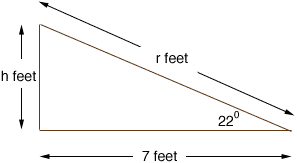Quandaries and Queries Here's my question:   A right triangle (roof of a house)  has a base of 7 feet and a 22 degree angle.  What is the height of the roof and what is the hypotenuse of the triangle.   Thank you. Hi, I drew a diagram and labeled the height of the roof as h feet.From the diagram the tangent of 22o is h/7, and hence h = 7tan(22o) = 70.4040 = 2.83 feet, which is very close to 2 feet 10 inches. If the hypotenuse of the triangle is of length r feet then Pythagoras' theorem tell us that r2 = 72 + h2 = 49 + 2.832 = 57.00 feet squares thus r = sqrt(57.00) = 7.55 feet, which is approximately 7 feet 6 and 5/8 inches. Penny Go to Math Central